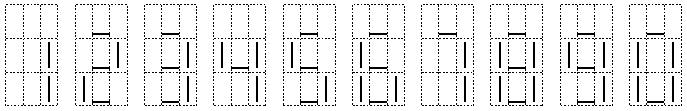Not an official ACM page
[Problem C | 1995 Western Europe problem set | Ed's programming contest problem archive | my home page]

# Problem B

## Bank (Not Quite O.C.R.)

Banks, always trying to increase their profit, asked their computer experts to come up with a system that can read bank cheques; this would make the processing of cheques cheaper. One of their ideas was to use optical character recognition (ocr) to recognize bank accounts printed using 7 line-segments.

Once a cheque has been scanned, some image processing software would convert the horizontal and vertical bars to ASCII bars '|' and underscores '_'.

The ASCII 7-segment versions of the ten digits look like this:A bank account has a 9-digit account number with a checksum. For a valid account number, the following equation holds: (d1 + 2 × d2 + 3 × d3 + . . . + 9 × d9) mod 11 = 0. Digits are numbered from right to left like this: d9d8d7d6d5d4d3d2d1.

Unfortunately, the scanner sometimes makes mistakes: some line-segments may be missing. Your task is to write a program that deduces the original number, assuming that:

• when the input represents a valid account number, it is the original number;
• at most one digit is garbled;
• the scanned image contains no extra segments.

For example, the following input

```    _  _     _  _  _  _  _
| _| _||_||_ |_   ||_||_|
||_  _|  | _||_|  ||_| _|
```
used to be "123456789".

### Input Specification

The input file starts with a line with one integer specifying the number of account numbers that have to be processed. Each account number occupies 3 lines of 27 characters.

### Output Specification

For each test case, the output contains one line with 9 digits if the correct account number can be determined, the string "`failure`" if no solutions were found and "`ambiguous`" if more than one solution was found.

### Example Input

```4
_  _     _  _  _  _  _
| _| _||_||_ |_   ||_||_|
||_  _|  | _||_|  ||_| _|
_  _  _  _  _  _     _
|_||_|| || ||_   |  |  ||
| _||_||_||_|  |  |  | _|
_  _  _  _  _  _  _  _  _
|_||_||_||_||_||_||_||_||_|
|_||_||_||_||_||_||_||_||_|
_     _  _  _  _  _  _  _
|_|  ||_||_||_||_||_||_||_|
|_|  ||_||_||_||_||_||_||_|
```

### Example Output

```123456789
ambiguous
failure
878888888
```